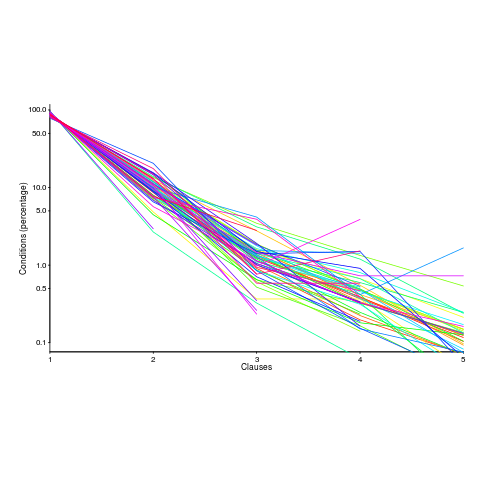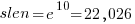## How should involved if-statement conditionals be structured?

Which of the following two if-statements do you think will be processed by readers in less time, and with fewer errors, when given the value of `x`, and asked to specify the output?

```// First - sequence of subexpressions
if (x > 0 && x < 10 || x > 20 && x < 30)
print("a");
else
print "b");

// Second - nested ifs
if (x > 0 && x < 10)
print("c");
else if (x > 20 && x < 30)
print("d");
else
print("e");
```

Ok, the behavior is not identical, in that the `else if`-arm produces different output than the preceding `if`-arm.

The paper Syntax, Predicates, Idioms — What Really Affects Code Complexity? analyses the results of an experiment that asked this question, including more deeply nested if-statements, the use of negation, and some for-statement questions (this post only considers the number of conditions/depth of nesting components). A total of 1,583 questions were answered by 220 professional developers, with 415 incorrect answers.

Based on the coefficients of regression models fitted to the results, subjects processed the nested form both faster and with fewer incorrect answers (code+data). As expected performance got slower, and more incorrect answers given, as the number of intervals in the if-condition increased (up to four in this experiment).

I think short-term memory is involved in this difference in performance; or at least I can concoct a theory that involves a capacity limited memory. Comprehending an expression (such as the conditional in an if-statement) requires maintaining information about the various components of the expression in working memory. When the first subexpression of `x > 0 && x < 10 || x > 20 && x < 30` is false, and the subexpression after the `||` is processed, there is no now forget-what-went-before point like there is for the nested if-statements. I think that the single expression form is consuming more working memory than the nested form.

Does the result of this experiment (assuming it is replicated) mean that developers should be recommended to write sequences of conditions (e.g., the first if-statement example) about as:

```if (x > 0 && x < 10)
print("a");
else if (x > 20 && x < 30)
print("a");
else
print("b");
```

Duplicating code is not good, because both arms have to be kept in sync; ok, a function could be created, but this is extra effort. As other factors are taken into account, the costs of the nested form start to build up, is the benefit really worth the cost?

Answering this question is likely to need a lot of work, and it would be a more efficient use of resources to address questions about more commonly occurring conditions first.

A commonly occurring use is testing a single range; some of the ways of writing the range test include:

```if (x > 0 && x < 10) ...

if (0 < x && x < 10) ...

if (10 > x && x > 0) ...

if (x > 0 && 10 > x) ...
```

Does one way of testing the range require less effort for readers to comprehend, and be more likely to be interpreted correctly?

There have been some experiments showing that people are more likely to give correct answers to questions involving information expressed as linear syllogisms, if the extremes are at the start/end of the sequence, such as in the following:

```     A is better than B
B is better than C
```

and not the following (which got the lowest percentage of correct answers):

```     B is better than C
B is worse than A
```

Your author ran an experiment to find out whether developers were more likely to give correct answers for particular forms of range tests in if-conditions.

Out of a total of 844 answers, 40 were answered incorrectly (roughly one per subject; it was a paper and pencil experiment, so no timings). It's good to see that the subjects were so competent, but with so few mistakes made the error bars are very wide, i.e., too few mistakes were made to be able to say that one representation was less mistake-prone than another.

I hope this post has got other researchers interested in understanding developer performance, when processing if-statements, and that they will be running more experiments help shed light on the processes involved.

## Growth of conditional complexity with file size

Conditional statements are a fundamental constituent of programs. Conditions are driven by the requirements of the problem being solved, e.g., if the water level is below the minimum, then add more water. As the problem being solved gets more complicated, dependencies between subproblems grow, requiring an increasing number of situations to be checked.

A condition contains one or more clauses, e.g., a single clause in: `if (a==1)`, and two clauses in: `if ((x==y) && (z==3))`; a condition also appears as the termination test in a for-loop.

How many conditions containing one clause will a 10,000 line program contain? What will be the distribution of the number of clauses in conditions?

A while back I read a paper studying this problem (“What to expect of predicates: An empirical analysis of predicates in real world programs”; Google currently not finding a copy online, grrr, you will have to hassle the first author: durelli@icmc.usp.br, or perhaps it will get added to a list of favorite publications {be nice, they did publish some very interesting data}) it contained a table of numbers and yesterday my analysis of the data revealed a surprising pattern.

The data consists of SLOC, number of files and number of conditions containing a given number of clauses, for 63 Java programs. The following plot shows percentage of conditionals containing a given number of clauses (code+data):The fitted equation, for the number of conditionals containing a given number of clauses, is:where:(the coefficient for the fitted regression model is 0.56, but square-root is easier to remember),, andis the number of clauses.

The fitted regression model is not as good whenoris always used.

This equation is an emergent property of the code; simply merging files to increase the average length will not change the distribution of clauses in conditionals.

When, all conditionals contain the same number of clauses, off to infinity. For the 63 Java programs, the meanwas 2,625, maximum 11,710, and minimum 172.

I was expecting SLOC to have an impact, but was not expecting number of files to be involved.

What grows with SLOC? Number of global variables and number of dependencies. There are more things available to be checked in larger programs, and an increase in dependencies creates the need to perform more checks. Also, larger programs are likely to contain more special cases, which are likely to involve checking both general and specific values (i.e., more clauses in conditionals); ok, this second sentence is a bit more arm-wavy than the first. The prediction here is that the percentage of global variables appearing in conditions increases with SLOC.

Chopping stuff up into separate files has a moderating effect. Since I did not expect this, I don’t have much else to say.

This model explains 74% of the variance in the data (impressive, if I say so myself). What other factors might be involved? Depth of nesting would be my top candidate.

Removing non-if-statement related conditionals from the count would help clarify things (I don’t expect loop-controlling conditions to be related to amount of code).

Two interesting data-sets in one week, with 10-days still to go until Christmas